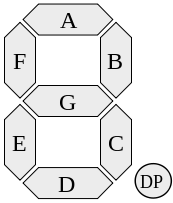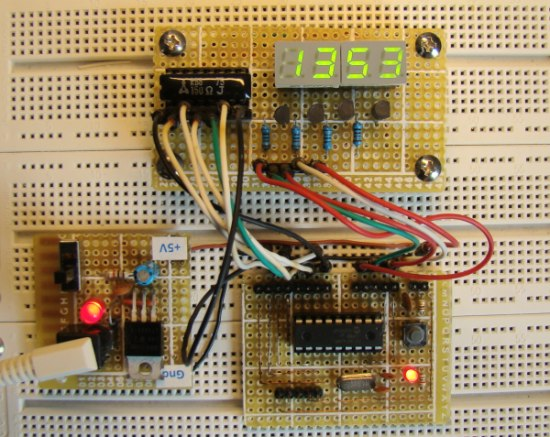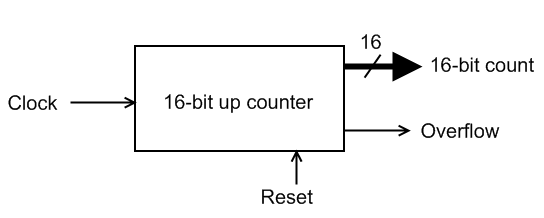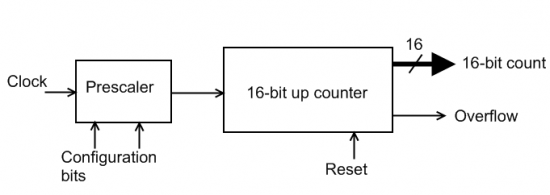## Netduino Day 3 – Multiplexed Seven-Segment LED displaysA visual output always adds value to any project. In this third day tutorial, we are going to display some numeric, alphabetic as well as two symbolic characters on a 4-digit seven segment LED module. The way we are interfacing the seven segments LEDs is known as multiplexing, which allows to save some I/O pins of Netduino as compared to driving them individually. The seven segment LEDs are resource and power hungry, but we are going to do this experiment just to get our feet wet in the microcontroller or embedded world and to understand the basics of a seven segments LED displays.

## Lab 11: Multiplexing seven segment LED displaysIn Lab 6, we discussed about interfacing a seven segment LED display to a PIC microcontroller. The seven segments were driven individually through separate I/O pins of the microcontroller. If we do just like that then for 4 seven segment LED displays, 28 I/O pins will be required, which is quite a bit of resources and is not affordable by mid-range PIC microcontrollers. That’s why a multiplexing technique is used for driving multiple seven segment displays. This tutorial shows how to multiplex 4 common anode type seven segment LED displays with a PIC16F628A microcontroller.

## Lab 6: Seven segment displayIntroduction

Seven segment LED displays are often found in clock radios, VCRs, microwave ovens, toys and many other household items. They are primarily used to display decimal numbers but they can also display a few alphabets and other characters. This experiment describes interfacing a seven segment LED display to a PIC16F688 microcontroller. You will make a hexadecimal counter that counts from 0 (00h) to 15 (0Fh) and display the value on the seven segment LED display.

Required Theory

A seven segment LED display is an special arrangement of 7 LED elements to form a rectangular shape using two vertical segments on each side with one horizontal segment on the top, middle, and bottom. By individually turning the segments on or off, numbers from 0 to 9 and some letters can be displayed. Seven segment displays sometime also have an eighth segment to display the decimal point. Therefore, a seven-segment display will require seven outputs from the microcontroller to display a number, and one more output if the decimal point is to be displayed too.

The segments are marked with non-capital letters: a, b, c, d, e, f, g and dp, where dp is the decimal point. The 8 LEDs inside the display can be arranged with a common cathode or common anode configuration. With a common cathode display, the cathodes of all the segment LEDs are tied together and this common point must be connected to the ground. A required LED segment is then turned on by applying a logic 1 to its anode. In common anode displays, all the anodes are tied together and the common anode is connected to the supply voltage Vcc. Individual segments are turned on by applying logic 0 to their cathodes.

When more than one seven segment display is used, a multiplexing technique is used to minimize the required number of microcontroller pins. We will discuss about that technique later.

In this experiment, a LT543 model common cathode seven segment display is used. The segment LEDs glow red when turned on. The module has 10 pins whose configuration is shown below.

## Timers and countersIntroduction

Most microcontrollers are equipped with one or more precision timing systems that can be used to perform a variety of precision timer functions including generating events at specific times, determining the duration between two events, or counting events. Example applications that require generating events include generating an accurate 1 Hz signal in a digital watch, keeping a traffic light green for a specific duration, or communicating bits serially between devices at a specific rate, etc.

Theory of operation

The main component of such a timing system is a free running binary counter. The counter increments for each incoming timing pulse. The counter counts continuously from 0 to 2n-1, where n is the number of bits in the counter. Since it runs independently, it can count inputs or clock pulses concurrently while the microcontroller is executing the main program. If the input clock to the binary counter has a fixed known frequency, we can make an accurate measurement of time interval by counting the pulses from the clock. For example, if a particular clock’s frequency is 1 MHz (period 1 µs), and we have counted 3000 pulses on the clock signal, then the elapsed time is 3000 microseconds.

In microcontrollers, the input clock to the internal timing system is software selectable. The microcontroller’s own clock source (which is fixed) can be selected as the clock input to the free running counter module of the timing system. In such a case, the timing system is known to be working as a timer because it is driven by a fixed known frequency clock. However, the input clock to the timing system can also be provided through an external I/O pin of the microcontroller in which case it is known to be working as a counter, and will count the external pulses which may appear at random intervals. These pulses could be manual inputs from a push button, or more likely, would be produced by some other signal source, like a road sensor that generates a pulse every time a car is passed over it.

A functional structure of a simple timer module is shown below. This has a free running 16-bit up counter that increments its value on each clock pulse. Thus, the 16-bit output count represents the number of pulses arrived since the counter was last reset to zero. We can convert this value into time interval by knowing the frequency (or period) of the input clock signal.Suppose, we want to measure the time elapsed between any two successive events. Lets assume that when the first event occurs, the timer is reset to zero, and when the second event occurs, the timer output is 25000. If we know that the input clock has period of 1 µs, then the time elapsed between the two events is 25000×1 µs = 25 milliseconds.

Since this timer’s counter can count from 0-65535 (216-1), this particular arrangement can measure time ranging from 0 to 65535 x 1 µs = 65.535 milliseconds, with a resolution of 1 µs. The maximum time interval a timer can measure is known as the timer’s range, whereas the resolution of a timer defines the minimum interval it can measure.

The timer module has an additional output, overflow flag, that indicates the counter reaches its maximum output, in which case the timer rolls over to 0. In microcontrollers, the built-in timers generate timer overflow interrupts when they roll over back to 0 after reaching their maximum counting range. We can count the number of times the timer overflows, and thus extend the range of time we can measure. In such a case,

elapsed time = (stop count – start count) + number of overflows x (2n-1),

where, n = 16 for a 16-bit counter.

A more advanced timer has a prescaler (shown below). A prescaler is essentially a configurable clock-divider circuit. Depending on the selected configuration bits, the prescaler output could be the same as the input signal, or it may have half the frequency, one-fourth the frequency, one-eighth the frequency, etc. Thus, a prescaler can extend a timer’s range by reducing its resolution. For example, if we use a prescaler to our previous 16-bit timer to divide the clock frequency by 8, then the new range of the timer will be 65535 x 8 µs = 524.280 milliseconds. But the new resolution is reduced to 8 µs.Timers in microcontrollers have additional configurable features such as enabling or stopping the counter, enabling or disabling interrupt generation when the counter overflows, selecting the clock edge for incrementing the counter, etc.